FreeRDP
Appender.c File Reference
`#include <winpr/config.h>`
`#include "Appender.h"`

## Functions

void WLog_Appender_Free (wLog *log, wLogAppender *appender)

wLogAppender * WLog_GetLogAppender (wLog *log)

BOOL WLog_OpenAppender (wLog *log)

BOOL WLog_CloseAppender (wLog *log)

static wLogAppender * WLog_Appender_New (wLog *log, DWORD logAppenderType)

BOOL WLog_SetLogAppenderType (wLog *log, DWORD logAppenderType)

BOOL WLog_ConfigureAppender (wLogAppender *appender, const char *setting, void *value)

## ◆ WLog_Appender_Free()

 void WLog_Appender_Free ( wLog * log, wLogAppender * appender )

WinPR: Windows Portable Runtime WinPR Logger

Licensed under the Apache License, Version 2.0 (the "License"); you may not use this file except in compliance with the License. You may obtain a copy of the License at

```http://www.apache.org/licenses/LICENSE-2.0
```

Unless required by applicable law or agreed to in writing, software distributed under the License is distributed on an "AS IS" BASIS, WITHOUT WARRANTIES OR CONDITIONS OF ANY KIND, either express or implied. See the License for the specific language governing permissions and limitations under the License.

Here is the call graph for this function: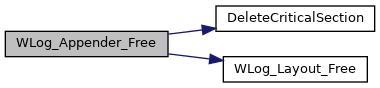Here is the caller graph for this function: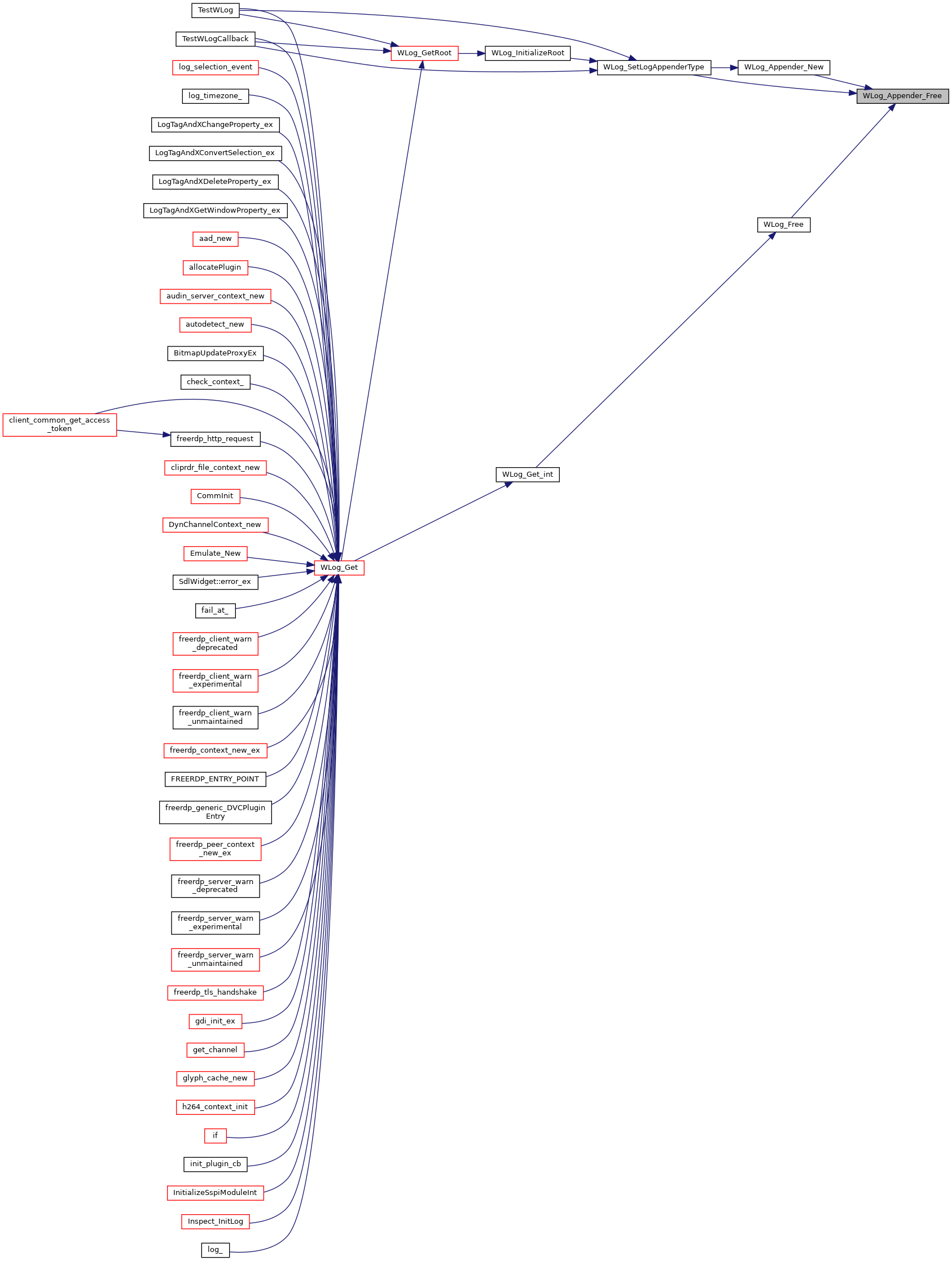## ◆ WLog_Appender_New()

 static wLogAppender* WLog_Appender_New ( wLog * log, DWORD logAppenderType )
static
Here is the call graph for this function: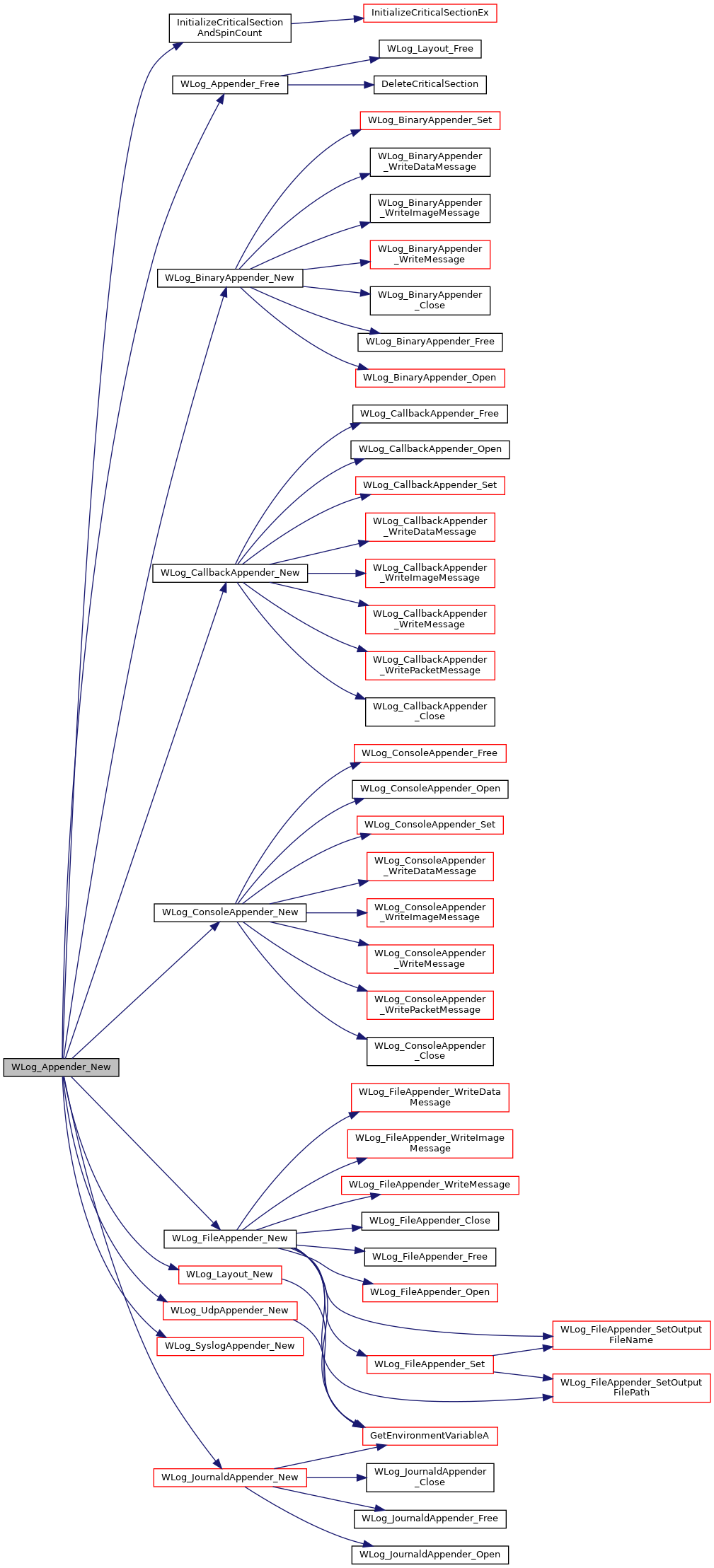Here is the caller graph for this function: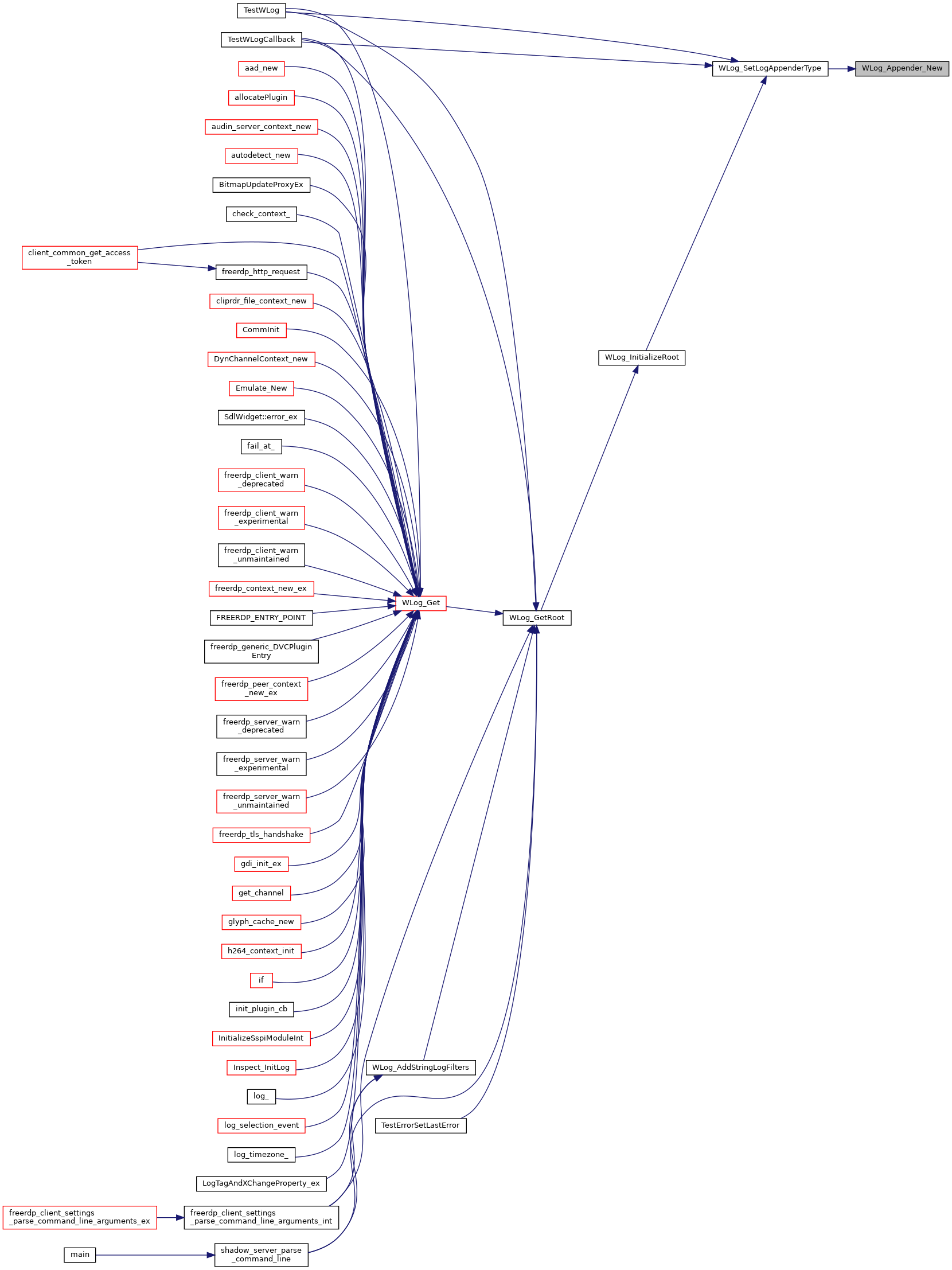## ◆ WLog_CloseAppender()

 BOOL WLog_CloseAppender ( wLog * log )
Here is the call graph for this function: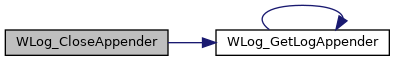Here is the caller graph for this function: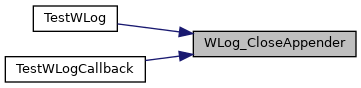## ◆ WLog_ConfigureAppender()

 BOOL WLog_ConfigureAppender ( wLogAppender * appender, const char * setting, void * value )
Here is the call graph for this function: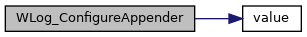Here is the caller graph for this function:## ◆ WLog_GetLogAppender()

 wLogAppender* WLog_GetLogAppender ( wLog * log )
Here is the call graph for this function: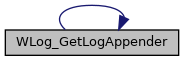Here is the caller graph for this function: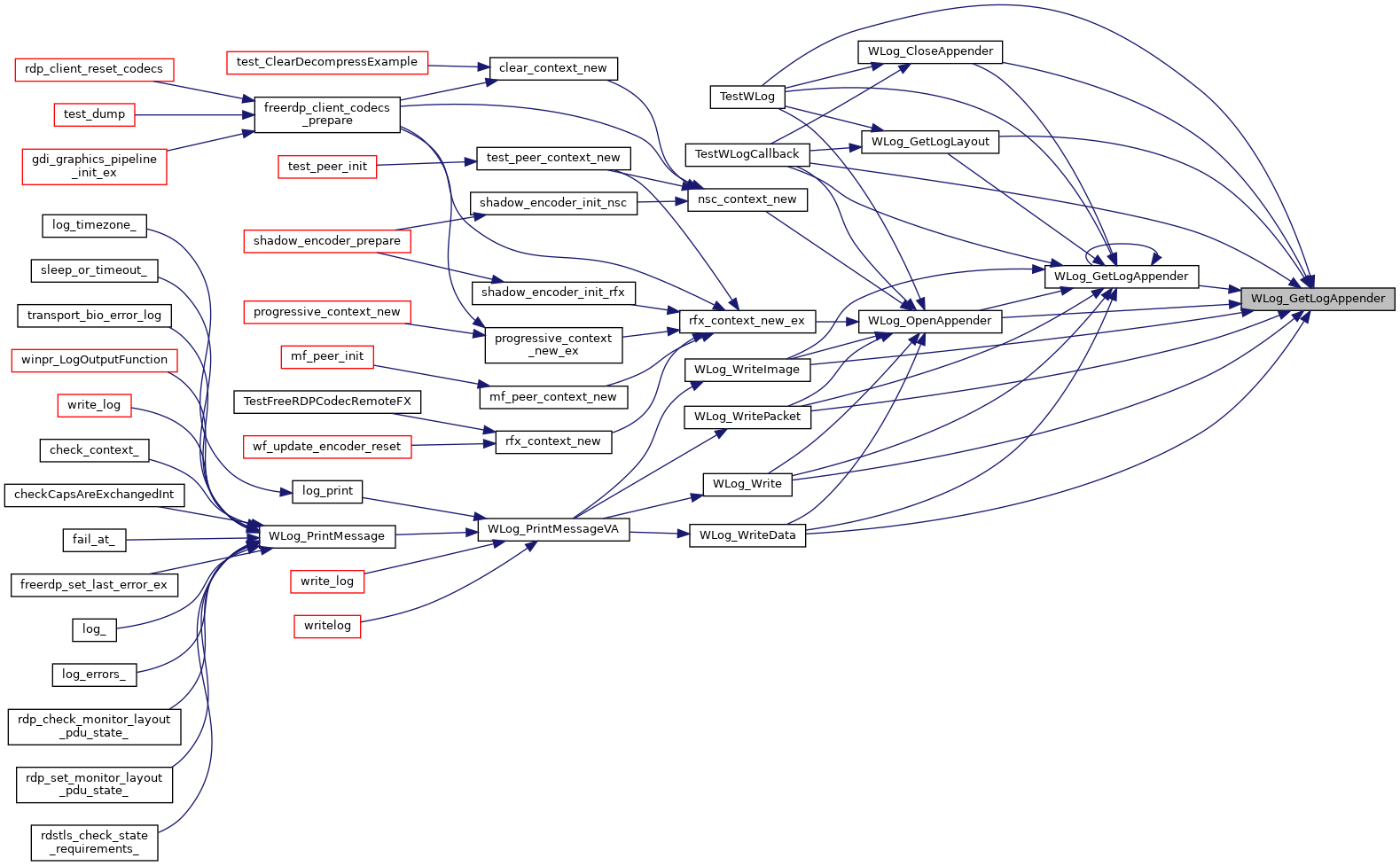## ◆ WLog_OpenAppender()

 BOOL WLog_OpenAppender ( wLog * log )
Here is the call graph for this function: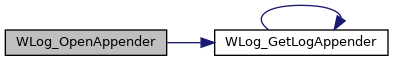Here is the caller graph for this function: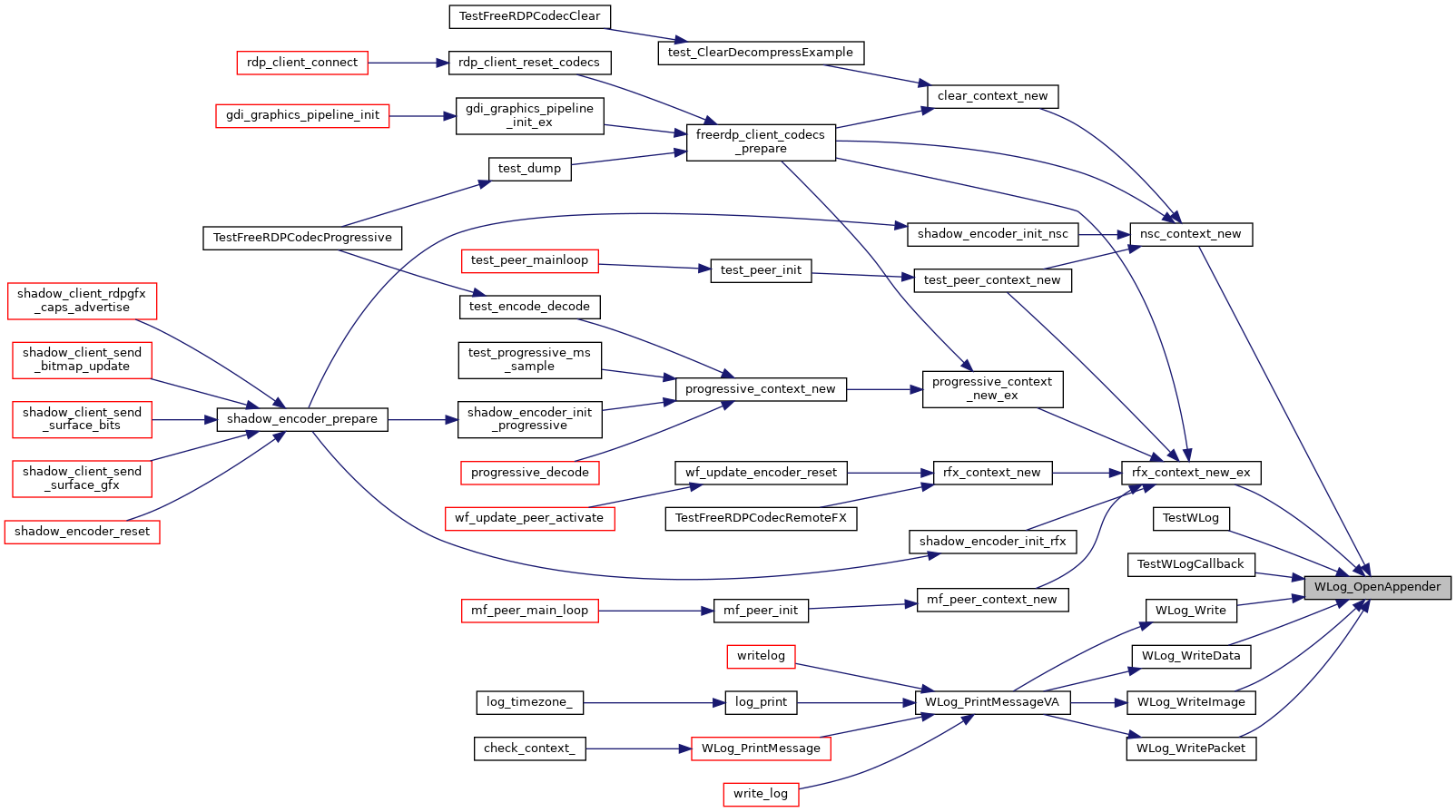## ◆ WLog_SetLogAppenderType()

 BOOL WLog_SetLogAppenderType ( wLog * log, DWORD logAppenderType )
Here is the call graph for this function: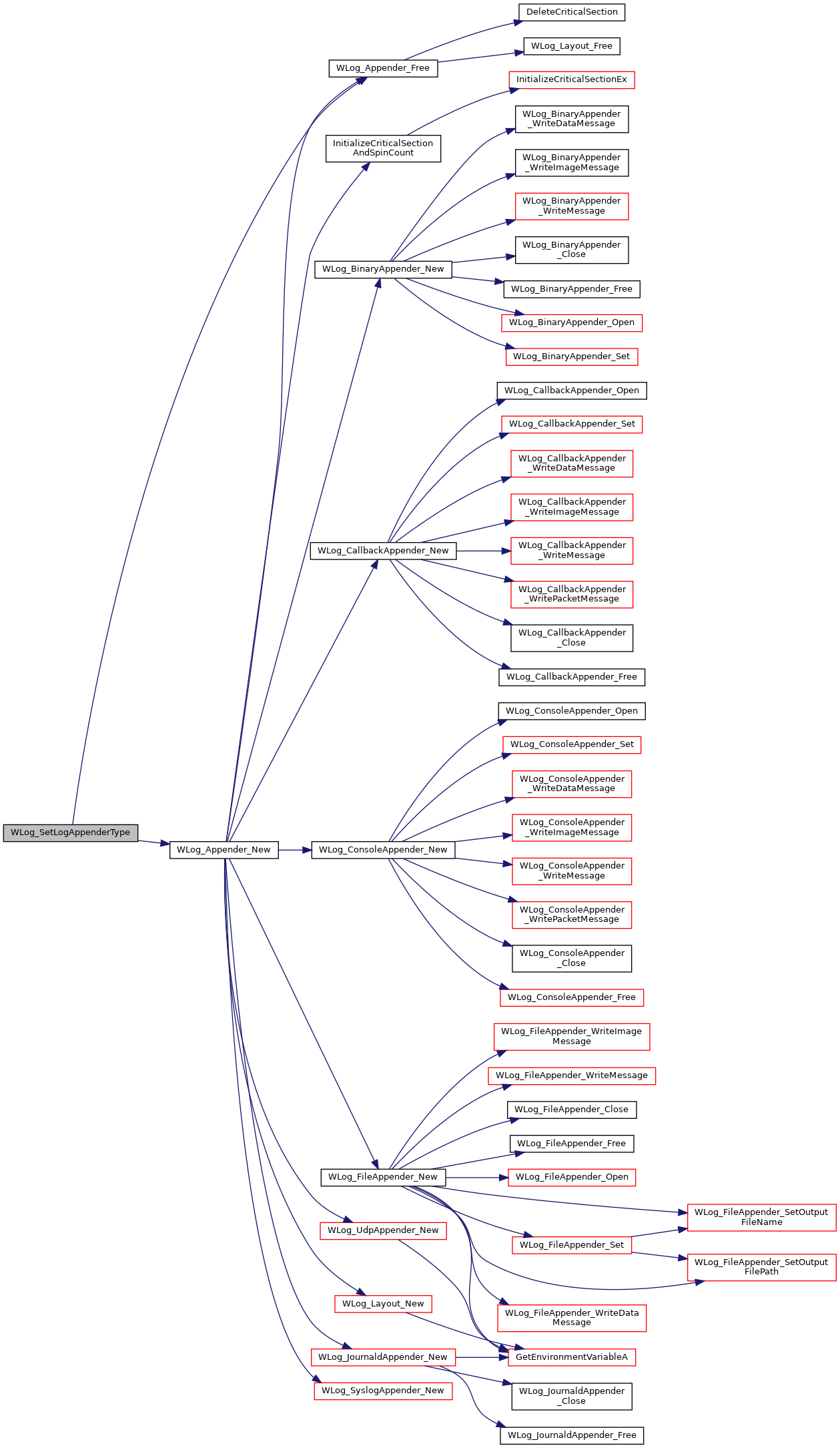Here is the caller graph for this function: